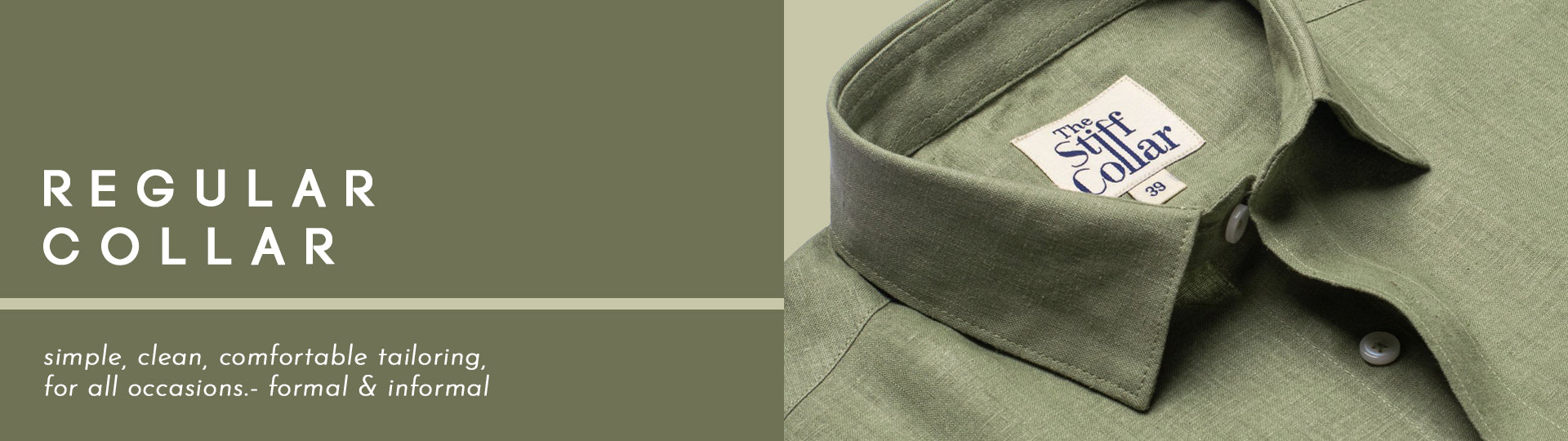Rs.150 cashback on orders over Rs.3000(Code PPD10).

# Formal ShirtsRs. 1,699.00

Rs. 1,899.00

Rs. 1,699.00

Rs. 1,499.00

Rs. 1,299.00

Rs. 1,499.00

Rs. 1,499.00

Rs. 1,499.00

Rs. 1,299.00

Rs. 1,499.00

Rs. 1,299.00

Rs. 1,499.00

Rs. 1,299.00

Rs. 1,299.00

Rs. 1,499.00

Rs. 1,499.00

Rs. 1,499.00

Rs. 1,499.00

Rs. 1,299.00
Rs. 1,499.00
( 13 % OFF )

Rs. 1,699.00

Rs. 1,499.00

Rs. 1,299.00

Rs. 1,499.00

Rs. 1,399.00
Rs. 1,499.00
( 7 % OFF )

Rs. 1,699.00

Rs. 1,299.00

Rs. 1,499.00

Rs. 1,499.00

Rs. 1,499.00
Rs. 1,799.00
( 17 % OFF )

Rs. 1,799.00

Rs. 1,799.00

Rs. 1,499.00

Rs. 1,499.00

Rs. 1,499.00

Rs. 1,699.00

Rs. 1,499.00

Rs. 1,499.00

Rs. 1,049.00
Rs. 1,799.00
( 42 % OFF )

Rs. 1,049.00

Rs. 1,049.00

Rs. 1,549.00
Rs. 1,799.00
( 14 % OFF )

Rs. 999.00

Rs. 1,299.00
Rs. 1,699.00
( 24 % OFF )

Rs. 1,299.00

Rs. 1,899.00

Rs. 1,049.00